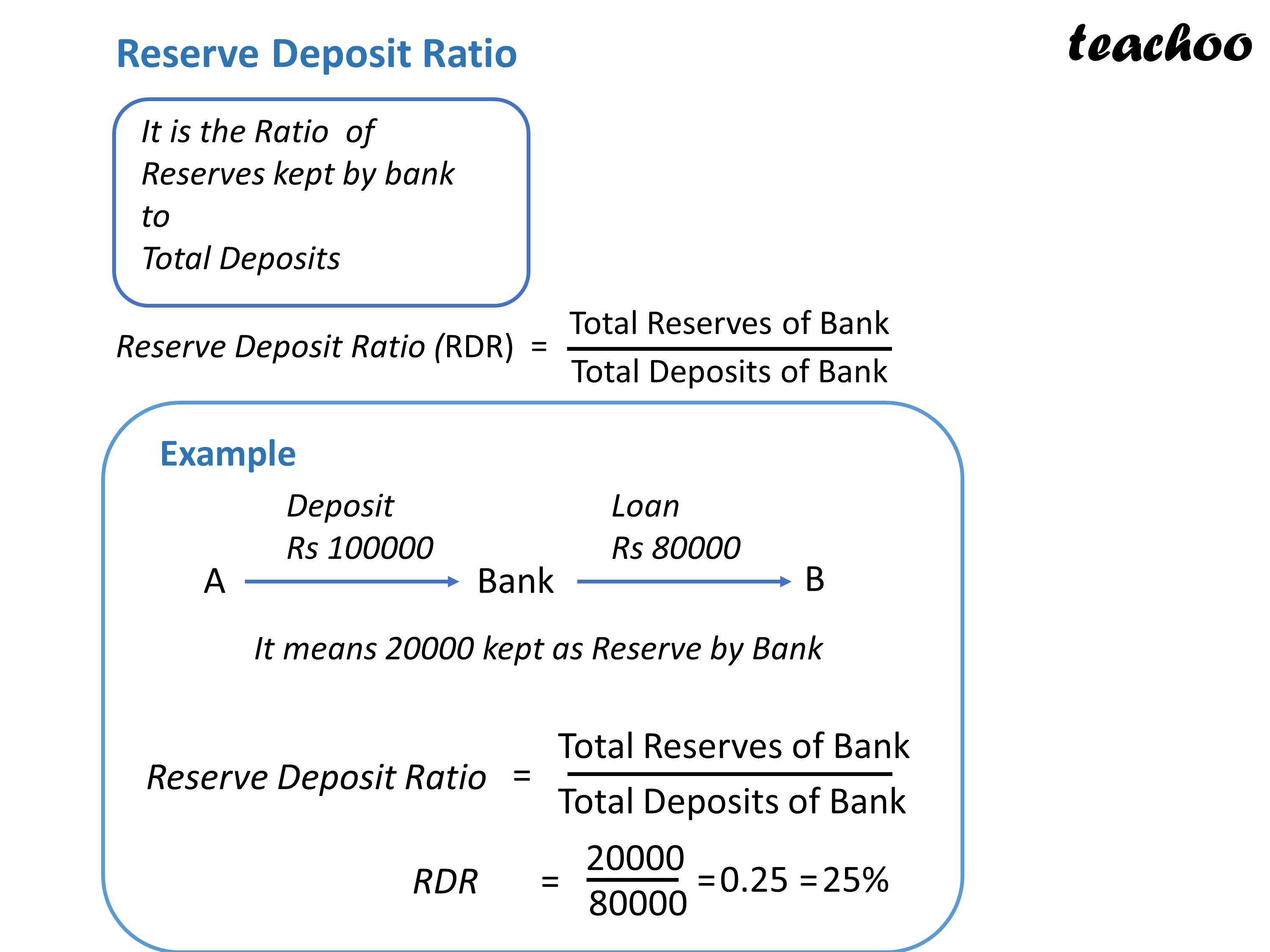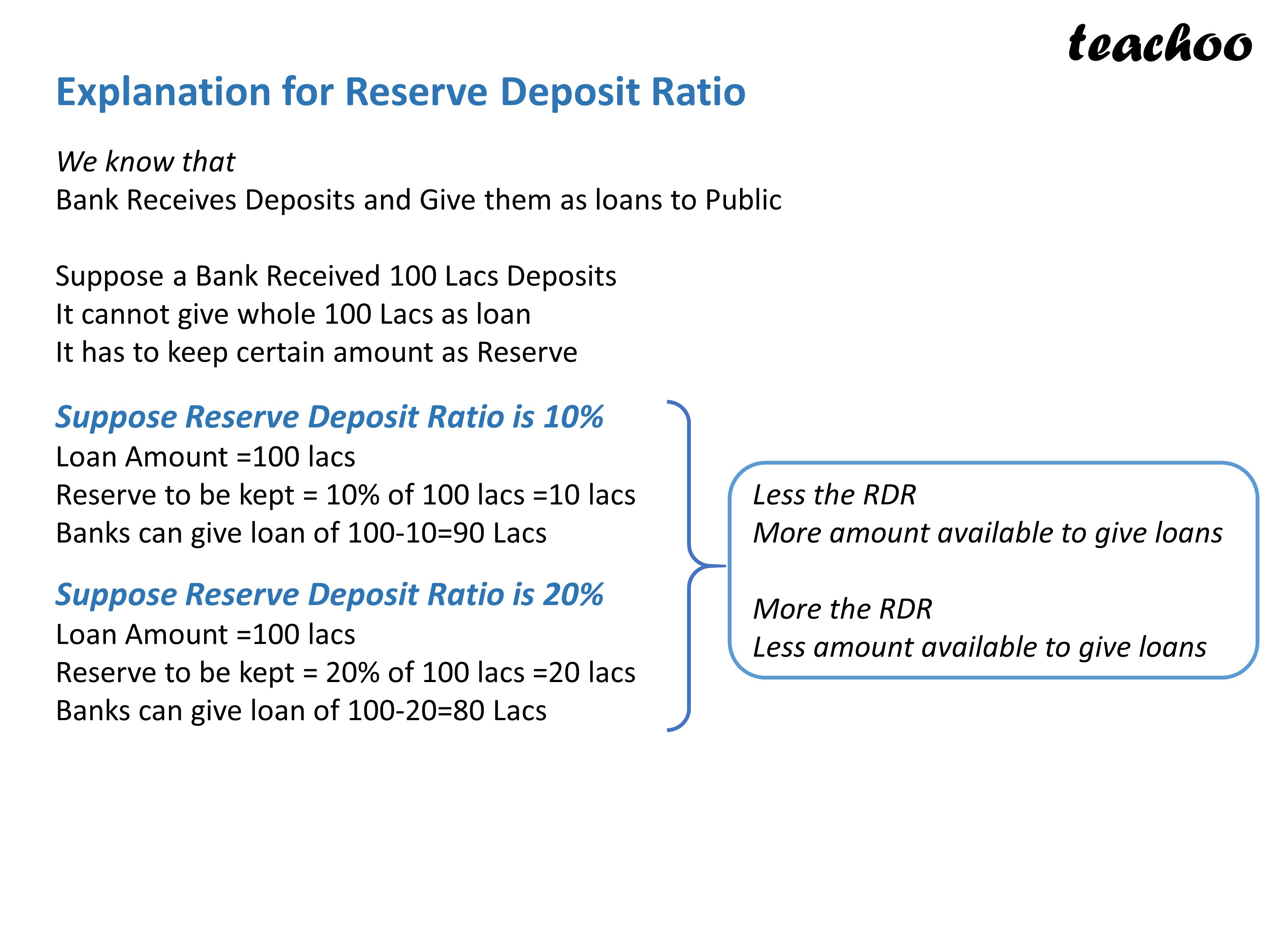Chapter 3 Part 2 - Banking

Economics Class 12
Macroeconomics

Important Ratios Used in Banking System

Here, we will learn about following Ratios

CDR (Currency Deposit Ratio)

RDR (Reserve Deposit Ratio)

SLR (Statutory Liquidity Ratio)

Also, we will learn about Bank RateLearn in your speed, with individual attention - Teachoo Maths 1-on-1 Class

### Transcript

Reserve Deposit Ratio It is the Ratio of Reserves kept by bank to Total Deposits Reserve Deposit Ratio (RDR) Total Reserves of Bank Total Deposits of Bank Example Loan Rs 80000 Deposit Rs 100000 It means 20000 kept as Reserve by Bank Reserve Deposit Ratio Total Reserves of Bank RDR 20000 80000 Explanation for Reserve Deposit Ratio We know that Bank Receives Deposits and Give them as loans to Public Suppose a Bank Received 100 Lacs Deposits It cannot give whole 100 Lacs as loan It has to keep certain amount as Reserve Suppose Reserve Deposit Ratio is 10% Loan Amount =100 lacs Reserve to be kept = 10% of 100 lacs =10 lacs Banks can give loan of 100-10=90 Lacs Suppose Reserve Deposit Ratio is 20% Loan Amount =100 lacs Reserve to be kept = 20% of 100 lacs =20 lacs Banks can give loan of 100-20=80 Lacs Less the RDR More amount available to give loans More the RDR Less amount available to give loans